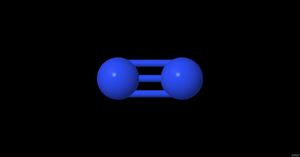Molecule
Name
Nitrogen
IUPAC name
Dinitrogen
Secondary names
Dinitrogen, Diatomic nitrogen, N2
InChI
InChI=1S/N2/c1-2
InChI key
IJGRMHOSHXDMSA-UHFFFAOYSA-N
CAS number
7727-37-9
natural $N_2$Formula
$N_2$
Chemical formula
N2
Structural formula
[N#N]
Charge
0
Unpaired electrons
0
Atom Name Number
$N$ Nitrogen 2
Relations
• no
Isotope mixture type
terrestrial abundance
Isotopes
Isotope Mole fraction
$^{14}N_2$ 0.9927332
$^{14}N^{15}N$ 0.0072535
$^{15}N_2$ 1.325e-05
Spin type
unique
Spin quantum numbers
0
Molar mass
28.01340642 $g/mol$
State STP
gas
Protic
aprotic
Polarity
nonpolar
Dipole
0.0 $D$
Molecule symmetry
Dinfh
Case
diatomic
Vibrations number
1
Vibrations
Label Mode Bond Symmetry Degeneracy Fundamental frequency Observed frequency Observed frequency Harmonic frequency Activity IR Activity Raman Strength Comments
V1 stretching $N\equiv N$ $\Sigma+$ no 2330.0 2358.57 inactive active ia vvw intensity: quadrupolar - Raman: 2331 $cm^{-1}$ (gas), 2329 $cm^{-1}$ (liq)
Vibrations of the main isotope: $^{14}N_2$. Data from HITRAN database and NIST Chemistry WebBook
$N\equiv N$ 1